## How to find the most common text value in a list in Google sheets?

If you have a long list of cells which contains some duplicate values, now, you want to find the most common text value which appears in the data list in Google sheets. How could you solve this job without checking one by one?

Find the most common value in a list in Google sheets with formula

Find the most common value in a list in Microsoft Excel with Kutools for Excel

#### Find the most common value in a list in Google sheets with formula

The following formula can help you to find the most common value in a list, please do as this:

Enter the following formula into a blank cell where you want to output the result, and then press Enter key, the most common value in the list will be displayed at once, see screenshot:

=ARRAYFORMULA(INDEX(A2:A17,MATCH(MAX(COUNTIF(A2:A17,A2:A17)),COUNTIF(A2:A17,A2:A17),0)))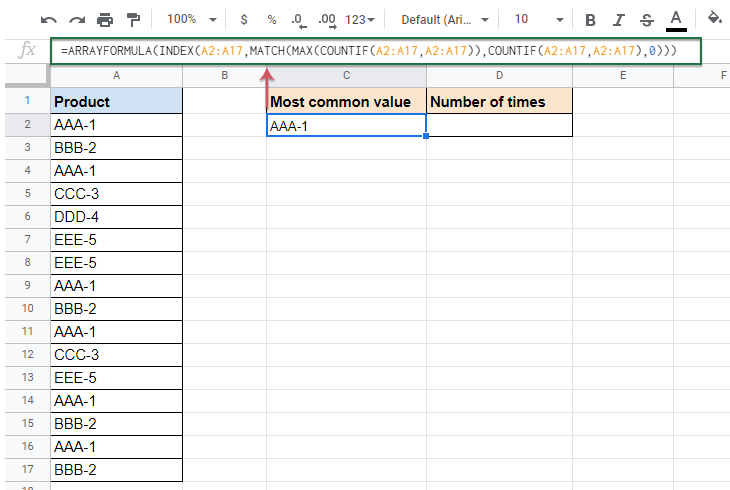To get the number of times which the most common value appears, you can apply this formula:

=ARRAYFORMULA(MAX(COUNTIF(A2:A17,A2:A17)))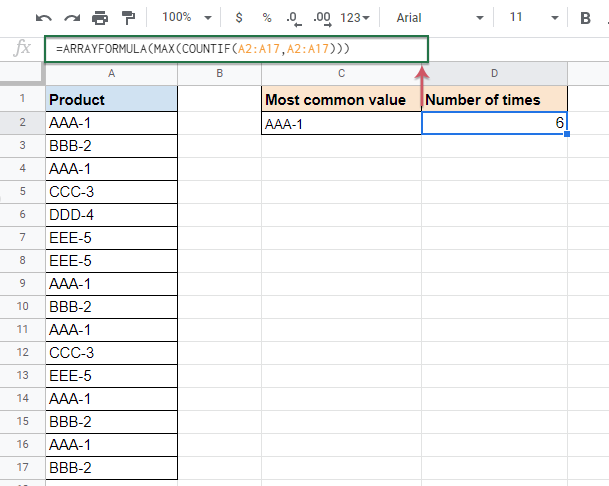Notes:

1. If you want to return the time occurence of each text, please apply the below formula into a blank cell, and then press Enter key to get the result:

=QUERY(A2:A17,"select A, count(A) where A is not null group by A order by count(A) DESC label A 'Product', count(A) 'Count'",-1)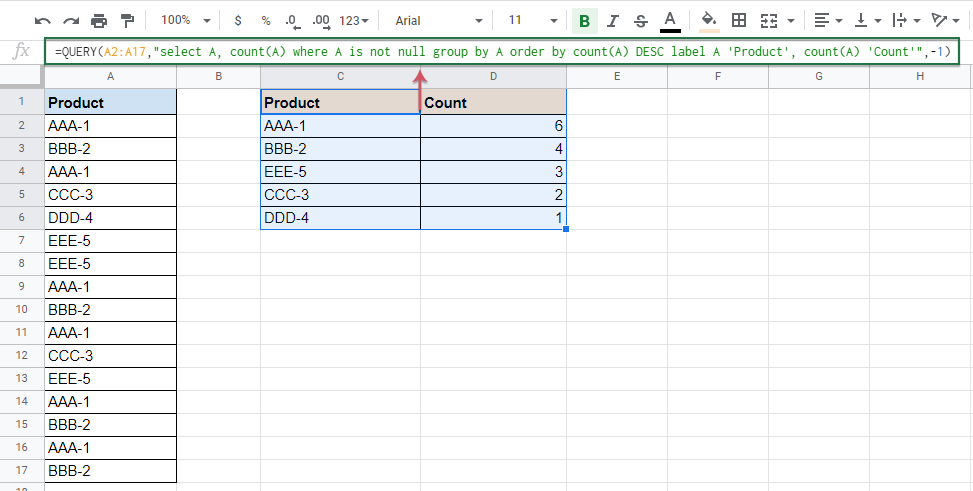2. In the above formulas, A2:A17 is the list of cells that you want to find the most common value from.

#### Find the most common value in a list in Microsoft Excel with Kutools for Excel

To find the most frequent value in a list in Excel worksheet, the Kutools for Excel’s Find most common value utility can help you without remebering any formulas.

Note:To apply this Find most common value, firstly, you should download the Kutools for Excel, and then apply the feature quickly and easily.

After installing Kutools for Excel, please do as this:

1. Click a blank cell where you want to output the result, and then click Kutools > Formula Helper > Formula Helper, see screenshot: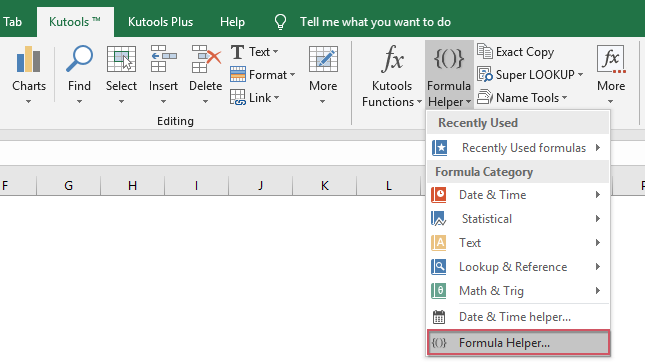2. In the Formulas Helper dialog box, do the following operations:

• Select Lookup option from the Formula Type drop down list;
• Then choose Find most common value from the Choose a fromula list box;
• In the right Arguments input section, select a list of cells that you want to extract the most common value beside the Range.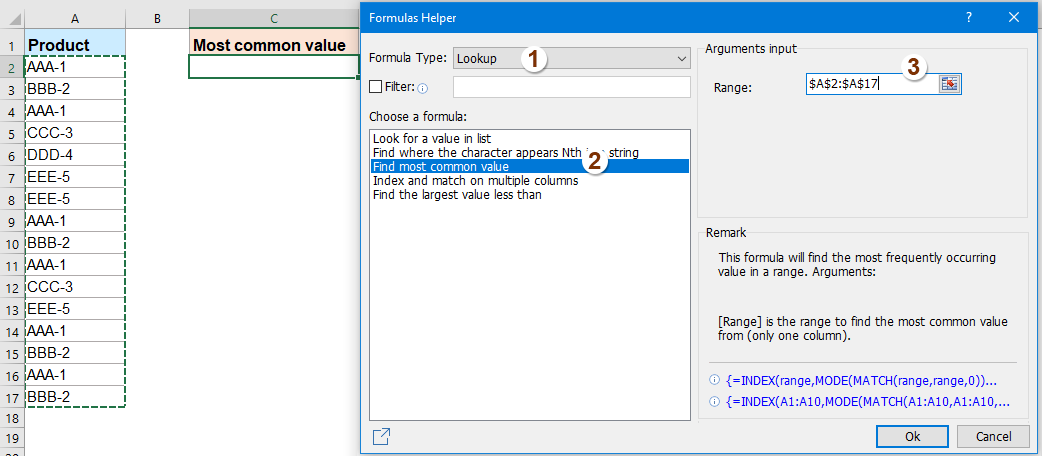3. And then click Ok button, the most common value has been displayed into the selected cell, see screenshot: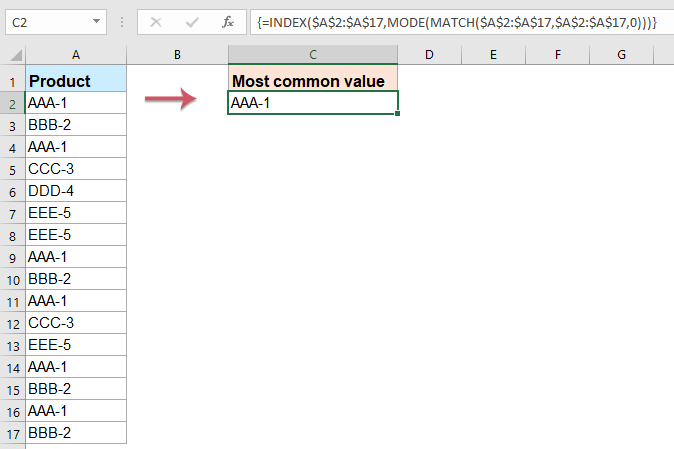#### More relative articles:

• Count Number Of Occurrence In A Column In Google Sheet
• Supposing, you have a list of names in Column A of Google sheet, and now, you want to count how many times each unique name is appeared as following screenshot shown. This tutorial, I will talk about some formulas for solving this job in Google sheet.

### Best Office Productivity Tools

 Popular Features: Find, Highlight or Identify Duplicates   |  Delete Blank Rows   |  Combine Columns or Cells without Losing Data   |   Round without Formula ... Super Lookup: Multiple Criteria VLookup  |   Multiple Value VLookup  |   VLookup Across Multiple Sheets   |   Fuzzy Lookup .... Advanced Drop-down List: Quickly Create Drop Down List   |  Dependent Drop Down List   |  Multi-select Drop Down List .... Column Manager: Add a Specific Number of Columns  |  Move Columns  |  Toggle Visibility Status of Hidden Columns  |  Compare Ranges & Columns ... Featured Features: Grid Focus   |  Design View   |   Big Formula Bar   |  Workbook & Sheet Manager   |  Resource Library (Auto Text)   |  Date Picker   |  Combine Worksheets   |  Encrypt/Decrypt Cells   |  Send Emails by List   |  Super Filter   |   Special Filter (filter bold/italic/strikethrough...) ... Top 15 Toolsets:  12 Text Tools (Add Text, Remove Characters, ...)   |   50+ Chart Types (Gantt Chart, ...)   |   40+ Practical Formulas (Calculate age based on birthday, ...)   |   19 Insertion Tools (Insert QR Code, Insert Picture from Path, ...)   |   12 Conversion Tools (Numbers to Words, Currency Conversion, ...)   |   7 Merge & Split Tools (Advanced Combine Rows, Split Cells, ...)   |   ... and more

Supercharge Your Excel Skills with Kutools for Excel, and Experience Efficiency Like Never Before. Kutools for Excel Offers Over 300 Advanced Features to Boost Productivity and Save Time.  Click Here to Get The Feature You Need The Most...#### Office Tab Brings Tabbed interface to Office, and Make Your Work Much Easier

• Enable tabbed editing and reading in Word, Excel, PowerPoint, Publisher, Access, Visio and Project.
• Open and create multiple documents in new tabs of the same window, rather than in new windows.
• Increases your productivity by 50%, and reduces hundreds of mouse clicks for you every day!No ratings yet. Be the first to rate!
This comment was minimized by the moderator on the site
If you're trying to accomplish this across multiple columns, just through the FLATTEN() function around the range of your multiple columns. For instance:
Your data is in column A, B, C and D, you'd put:
flatten(A2:D17)

Making the entire formula look like:
=ARRAYFORMULA(INDEX(flatten(A2:D17),MATCH(MAX(COUNTIF(flatten(A2:D17),flatten(A2:D17))),COUNTIF(flatten(A2:D17),flatten(A2:D17)),0)))

Cheers!
This comment was minimized by the moderator on the site
Hi,

How would I use this function but calculate only a subtotal of a filter.

I tried something like this but it didn't seem to work
=ARRAYFORMULA(INDEX(subtotal(101,A2:A17),MATCH(MAX(COUNTIF(subtotal(101,A2:A17,A2:A17))),COUNTIF(subtotal(101,A2:A17,A2:A17),0)))

Can't seem to figure it out and I've been searching the web for hours.

Thanks!
This comment was minimized by the moderator on the site
Hello. Thank you so much for posting this -- it was a huge help. How would the formula change if I wanted the 2nd most common, 3rd most common, etc value in a list? Thanks.
This comment was minimized by the moderator on the site
How do you exclude a particular value from the formula, such as —
There are no comments posted here yet